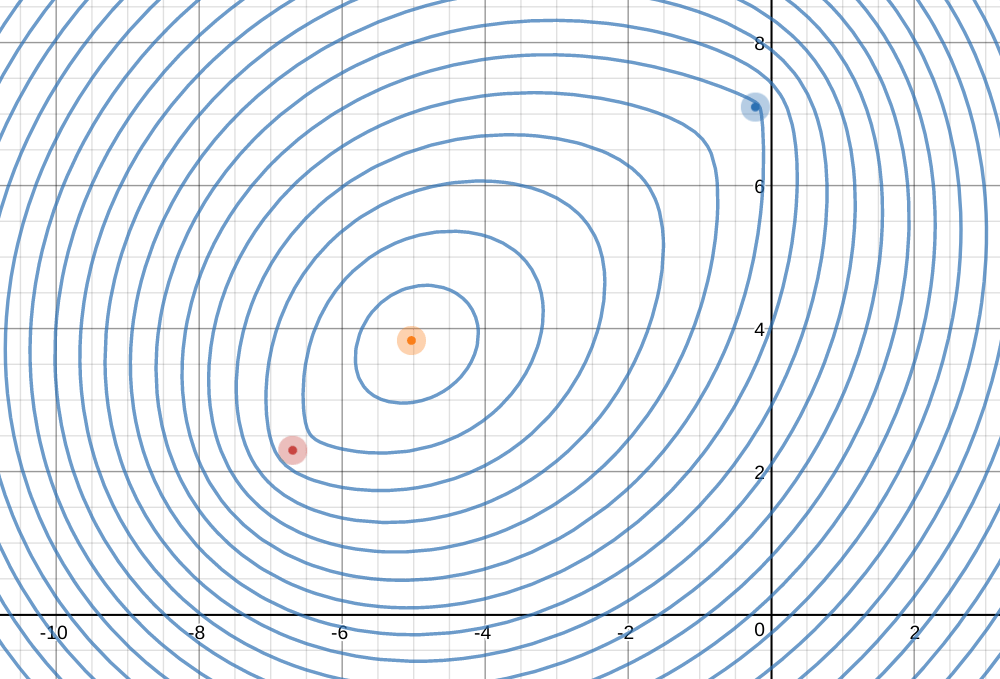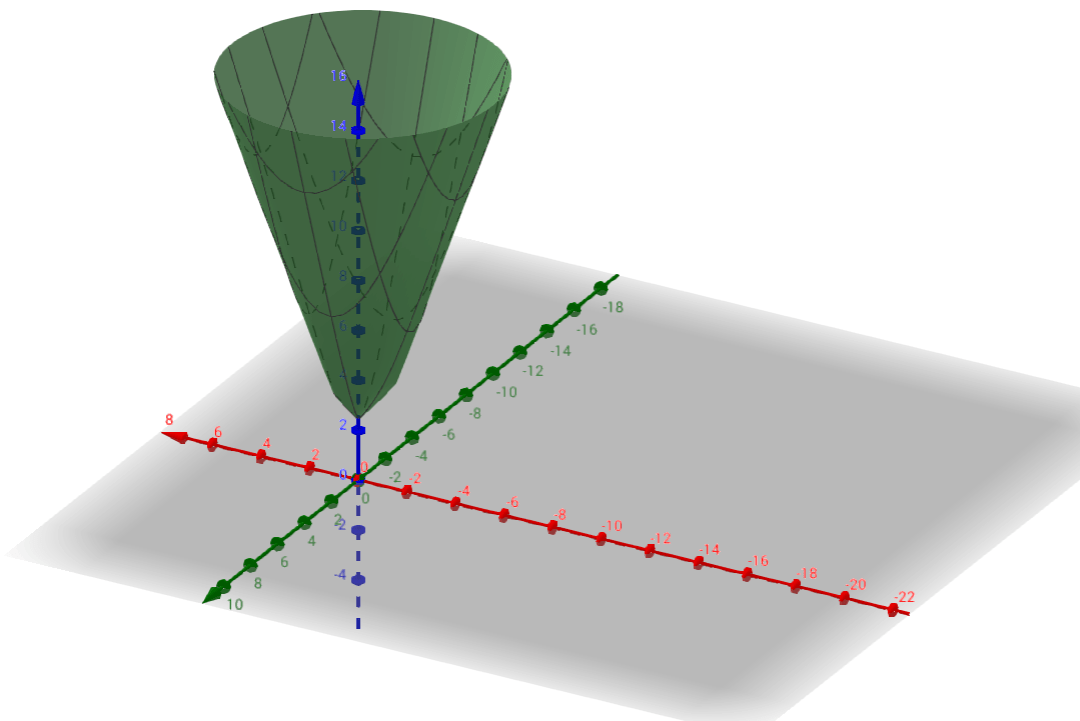# Generalizing Circles and Ellipses

[Reading and thinking about: n-Ellipses and the Minimum Distance Sum Problem, a paper that I was able to stick pretty well with until about the last third.]

## 1. Generalizing Circle Definitions

There often comes a point in my geometry classes where I ask students to come up with a definition that describes a circle. It’s always interesting to see the ideas they land on. Some students try to capture the perfect curviness of a circle; others talk about the circle’s symmetry. When I share the “textbook” definition (which kids rarely come up with) there are sometimes sighs of relief or yelps of excitement in the room:

Circle: Take all the points that are a certain distance away from a center point — that’s a circle.

It’s a beautifully simple definition, and it’s one that leaves plenty of room for generalization.

If we’re looking to generalize the circle, a great next step is to analogously define an ellipse. I like to imagine the center of a circle splitting in half and turning into the two foci of the ellipse.(Sorry for the glitchy gif.)

We can define an ellipse in a way that is analogous to the “textbook” definition of the circle:

Ellipse: Take all the set of points that are a certain distance away from two focal points — that’s an ellipse.

Just to clarify, what I mean by “certain distance away” is we’re looking for the sum of the distances to the two focal points. So if our “certain distance” is 10, then a point that is 3 away from one focal point and 7 away from the other would be part of the ellipse.

## 2. Generalizing Circle Constructions

Sometimes you see geometry problems about goats on a rope. The point being that the rope-stuck goat is restricted by a circle. That goat could trace out a circle, and you can also trace a circle with a pin, string and paper.  You can also make a lovely ellipse with two focal points with some string and some pins:Why stop here, though? The definition of ellipse (let’s call it a 2-ellipse) can be expanded to include 3 focal points, 4 focal points, 176 focal points, really focal points, no matter what integer is.

n-ellipse: Take all the points whose distances to each of n focal points sum to a certain number. This is the n-ellipse.

James Clerk Maxwell was sixteen years old when he invented a contraption that generalized the pin and string method for constructing an ellipse. He figured out a clever way to wrap the string around so that you could use pins and string to produce a 3-ellipse.From the collected papers of Maxwell. He presented this at the Royal Society of Edinburgh. The three Fs are the focal points.

## 3. Generalizing Equations

The equations generalize nicely too.

Circle (a.k.a. 1-Ellipse):$\sqrt{(x-a)^2+(y-b)^2} = D$2-ellipse:$\sqrt{(x-a_0)^2+(y-b_0)^2} + \sqrt{(x-a_1)^2+(y-b_1)^2} = D$3-ellipse:$\sqrt{(x-a_0)^2+(y-b_0)^2} + \sqrt{(x-a_1)^2+(y-b_1)^2} + \sqrt{(x-a_2)^2+(y-b_2)^2} = D$Alas, no tex.

n-ellipse:$\sum_{i=0}^{n}\sqrt{(x-a_i)^2+(y-b_i)^2} = D$

(Most students learn a different equation for an ellipse, defined in terms of minor and major axes. It’s not exactly a load of fun to analytically derive that from this equation for the 2-ellipse, I’ve found.)

## 4. Smallest Ellipses?

Each of the definitions above leave room for a “certain distance.” But are there any restrictions on what that distance can be?

For a circle, that “certain distance” is the radius, and the radius can be whatever magnitude that you like. Given a center, make your radius huge, make it tiny: there’s always a circle of those points just that distance away.For a 2-ellipse, though, this isn’t so. Imagine one focal point that’s one million miles away from the other, and imagine that we’re interested in the points whose sum of distances from the focal lengths is one inch. That’s simply impossible to achieve. Being one inch away from one focal point would necessarily involve being nearly a million miles away from the other.

At some point, an ellipse might be too small for its focal points.

So: what is that least distance, given two focal points? What is the least amount of distance that you can sum to, given focal points? What are these critical points of the distance sum function?

For a 2-ellipse, the minimum distance should just be the distance between the two focal points.But what about for a 3-ellipse? or a 4-ellipse? an n-ellipse?

I’m still having trouble with the analytic solution in that paper, but graphically you can just graph a bunch of different distances and try to see where things are headed. Here is a snapshot from this Desmos graph, which graphs a collection of distances for three foci (which you can move around the graph — try it, it’s fun!).This is a contour plot of the distance-sum function. We could also visualize this as slices of a 3D graph, where the “z” axis is the varying distance-sum.

Here is a 3D graph of a 2-ellipse, showing how it bottoms out at a very narrow ellipse (practically a line segment) at a height of 2 — the distance between the two foci:And here is a 3D graph of a 3-ellipse, which also bottoms out at a certain distance-sum above 0:In contrast, here’s the 1-ellipse, a circle. It has no minimum radius:## 5. A cool problem

Say that you have three cities that are special to you, for whatever reason. Maybe you grew up in one of them, went to college in another, and have some family in the third.

If your only priority was to be as close as possible to each city, where should you live?

This is really, really similar to the question of where the minimum distance-sum is for an n-ellipse.(It makes sense that it starts getting circle-ish as we get farther away from the focal points. From a million miles up in the air, those focal points might as well be on top of each other.)

Go to this graph and play around with your cities. You can also add more cities.

## 7. Now what?

I’m not sure. I tried to write this a few different ways, but in the end all I could figure out was the info dump you see above. Is there a way to rewrite this so that there’s a stronger narrative? Should I come up with problems for each section to give readers a chance to stop and think about stuff?

I think this needs to go on the back-burner, and I just need to write more about math and hope that eventually I figure a few more things out.